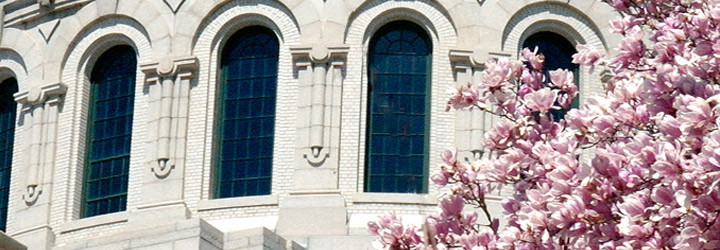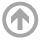Evelyn LunasinThe projects that I plan to expand on over the next few years concern the mathematical analysis of certain turbulence models and related equations. My research also involves the direct numerical simulation of these equations with applications to optimal stirring strategies for passive scalars and mixing, data assimilation, which is of practical interest to weather and climate prediction, image reconstruction, and nonlinear control theory with vast engineering applications.

Publications

As of 2018,  here are the papers I have published:

1. A. Farhat, E. Lunasin and E. S. Titi, A Data Assimilation Algorithm: The Paradigm of the 3D Leray-$\alpha$ Model of Turbulence, Nonlinear Partial Differential Equations Arising from Geometry and Physics, Cambridge University Press, London Mathematical Society, Lecture Notes Series.
2. E. Lunasin and E.S. Titi, Finite determining parameters feedback control for distributed nonlinear dissipative systems - a computational study, Evolution Equations and Control Theory, 6, no. 4  (2017), 535--557.
3. A. Farhat, E. Lunasin and E. S. Titi, On the Charney conjecture of data assimilation employing temperature measurements alone: The paradigm of 3D Planetary Geostrophic model, Mathematics of Climate and Weather Forecasting, 2, (2016), 61--74.
4. A. Farhat, E. Lunasin and E. S. Titi, Continuous data assimilation for a 2D Benard convection system through horizontal velocity measurements alone, J. Nonlinear Science,  2, (2016), 1065--1087.
5. A. Farhat, E. Lunasin and E. S. Titi, Data assimilation algorithm for 3D Benard convection in porous media employing only temperature measurements, Journal of Math. Anal. and App. 438 (1), (2016), 492 -- 506.
6. E. Lunasin, R. Malek-Madani and M. Slemrod, A dynamical systems approach to mathematical modeling of tornadoes, Bull. Inst. Math. Acad. Sinica 11 (1), (2016), 141--161.
7. A. Farhat, E. Lunasin and E.S. Titi, Abridged continuous data assimilation for the 2D Navier-Stokes equations utilizing measurements of only one component of the velocity field, Journal of Mathematical Fluid Mechanics 18 (1), (2016), 1--23.
8. A. Farhat, M.S. Jolly and E. Lunasin, Bounds on energy and enstrophy for the 3D Navier-Stokes-$\alpha$ and Leray-$\alpha$ models, Communications on Pure and Applied Analysis 13, (2014) 2127--2140.
9.  A. Larios, E. Lunasin and E.S. Titi, Global well-posedness for the 2D Boussinesq system without heat diffusion and with anisotropic viscosity, J. Differential Equations  255, (2013), 2636--2654.
10. E. Lunasin, Z. Lin, A. Novikov, A. Mazzucato and C. Doering, Optimal Stirring Strategies with finite energy, finite power or finite palenstrophy constraint,  Journal of Mathematical Physics 53, (2012), 1--15.
11. M. Ebrahimi, M. Holst and E. Lunasin, The Navier-Stokes Voight for image inpainting, IMA Journal of Applied Mathematics (2012), 1--26.
12. B. Wen, N. Dianati, E. Lunasin, G. Chini and C. Doering, New upper bounds and reduced dynamical modeling for Rayleigh-Benard convection in a fluid saturated porous layer, Comm. Nonlinear Science and Num. Simulations, 17 (5), (2011), 2191--2199.
13. M. Holst, E. Lunasin and G. Tsotgtgerel, Analytical study of generalized $\alpha$-models of turbulence, Journal of Nonlinear Science,  20 (5), (2010), 523--567.
14. E. Lunasin, S. Kurien and E.S. Titi, Spectral scaling of the Leray-$\alpha$ model for two-dimensional turbulence, Journal of Physics A: Math. Theor. 41, (2008), 344014.
15. E. Lunasin, S. Kurien, M. Taylor and E.S. Titi, A study of the Navier-Stokes-$\alpha$ model for two-dimensional turbulence,Journal of Turbulence,  8, (2007), 751--778.
16. Y. Cao, E. Lunasin, and E.S. Titi, Global well-posedness of viscous and inviscid simplified Bardina turbulence models, Communications in Mathematical Sciences 4, no. 4, (2006), 823--847.
17. A. Ilyin, E. Lunasin and E.S. Titi, A modified-Leray-$\alpha$ sub-grid scale model of turbulence, Nonlinearity 19, (2006), 879--897.

Ph.D. Thesis:  Analytical and numerical study of certain sub-grid scale $\alpha-$models of turbulence. University of California, Irvine, (2007).

Collaborators

My background in scientific computing and the mathematical theory of fluid dynamics has opened many doors to collaborate with researchers in numerous institutes.  Here is an incomplete list of current and past collaborators.

• Svetlana Avramov-Zamurovic, United States Naval Academy
• Cody Brownel, United States Naval Academy
• Charles Doering, University of Michigan
• Aseel Farhat, Florida State University
• Michael Holst, University of California, San Diego
• Athanasios Iliopoulos, U.S. Naval Research Laboratory
• Alexei Ilyin, Keldysh Institute of Applied Mathematics
• Michael Jolly, Indiana University
• Susan Kurien, Los Alamos National Laboratory